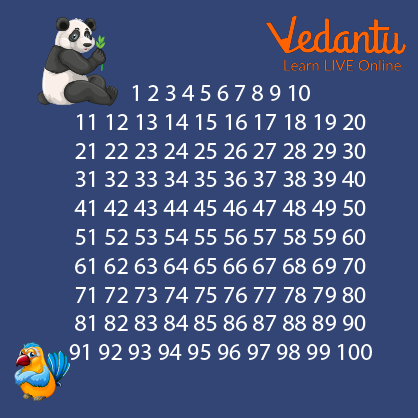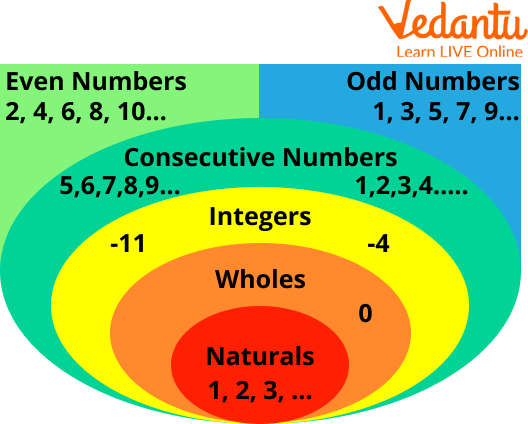Courses
Courses for Kids
Free study material
Free LIVE classes
More

# Hidden Numbers GamesLIVE
Join Vedantu’s FREE Mastercalss

## What is the Hidden Number?

A hidden number game is a lighthearted game that challenges your capacity to locate numbers within a context to help kids with their number sense. Finding lists of numbers within an unscrambled set of numbers scenario is a requirement for all hidden number games.

In the number games, we have to locate the precise number that is concealed in the table of numbers. Children must attentively read the question before locating the precise number from the number table and completing the blanks. Colour the number in the table red to indicate that it has been utilised. Let’s understand “what a hidden number is” in detail.

## Use of Hidden Numbers

For children to comprehend the sides, above, and below, the number table is quite helpful. Children can use this worksheet on number games to practise their understanding of the right side, left side, over, and below in actual situations. Take a printout of the number table, then have some time doing this worksheet. Now, it is clear what a hidden number is.

## Hidden Number Games

Children can observe how one is done for them to obtain a clear picture of what to do, for instance, and then follow the number table chart's instructions for colouring that number.

1) To identify the hidden number, read the following and highlight it in red in the number table below:Hidden Number Games

(a) above 55 45 (m) left of 69 68

(b) left of 53 52 (n) below 48 58

(c) right of 43 44 (o) right of 49 50

(d) below 32 42 (p) above 76 66

(e) above 86 76 (q) above 90 80

(f) right of 73 74 (r) left of 50 49

(g) below 60 70 (s) right of 53 54

(h) left of 57 56 (t) left of 63 62

(i) below 38 48 (u) below 36 46

(j) right of 59 60 (v) above 74 64

(k) below 62 72 (w) below 65 75

(l) right of 77 78 (x) right of 78 79

2) To identify the hidden number, Visualise the following image carefully and highlight the displayed number in redNumber of Games

The number hidden in the below-given image is 2 and two

## Numbers

A number is a fundamental building block of mathematics. Numbers are used for indexing, counting, measuring, and a variety of other tasks. Before using numbers, tally marks were first used.

## Types of Numbers

Consecutive Numbers: From the smallest number to the highest number, consecutive numbers follow one another. The typical difference between every two numbers is 1. For example, 3 and 4 are consecutive numbers.

Integers: Numbers made up of both whole numbers & negative numbers are known as integers. For example 1,0,2,-3,-4.

Whole Numbers and Natural Numbers: Whole numbers and natural numbers are both non-negative integers, zero also included. Whole numbers do not have any decimal or fractional components, it should be noted.

Even and Odd Numbers: Even numbers are precisely divisible by two and can be split into 2 equal groups or pairs. Whole numbers that cannot be split by two in full are referred to as odd numbers. For example, 2 is an even number while 7 is an odd number.Numbers

## Properties of Numbers

Commutative Property: Both addition and multiplication are compatible operations with this property of numbers.

Associative Property: Numbers have the associative property, which is relevant to both addition and multiplication.

Distributive Property: The sum of the products of each addend and the third number equals the combination of the sum of two numbers and the third number.

Identity property: We have a multiplicative identity of 1 and an additive identity of 0.

Inverse property: The identity can be obtained by applying an arithmetic operation to a number and its inverse.

## Summary

A number is a numerical unit of measurement and labelling in mathematics. Natural numbers are those numbers that begin with 1 and go all the way up to infinity. Whole numbers are those numbers that begin at zero. Positive and negative numbers, as well as zero, make up integers. Integers, fractions, and ratios are the components of rational numbers, which have the form p/q. Fractions and ratios are not used to express irrational numbers. Natural numbers, whole numbers, rational numbers, and irrational numbers are all types of real numbers.

Last updated date: 23rd Sep 2023
Total views: 110.7k
Views today: 3.10k

## FAQs on Hidden Numbers Games

1. What are the numerals known as?

More commonly, specific numbers can be symbolised using symbols known as numerals; for instance, the numeral "5" stands in for the number five. A numeral system is a way of writing numbers; it's a way of mathematically notating a collection of numbers by utilising a consistent set of digits or other symbols. The value of a numeral is the number it stands for. All modern numbers cannot be represented by all number systems; Roman numerals, for instance, do not have a zero.

2. Do we have names for numbers?

Like everything else in the universe, numbers have names that correspond to them. Numbers have names in mathematics. In the word format, the numbers from 1 to 100 are represented by the number names 1 to 100. Among the number names from 1 to 100, 98 are written as "Ninety-eight," 57 as "Fifty-seven," 45 as "Forty-five," and so forth. Depending on where the digits are positioned in a number, we can represent the positional value of those digits. To write the spellings of the numbers, we may simply consult the chart of number names from 1 to 100. Three hundred forty-nine is the word form of 349, which has the expanded form 300 + 40 + 9.

3. Who found zero?

"Zero and its operation are first defined by {Hindu astronomer and mathematician} Brahmagupta in 628," said Gobets. He developed a symbol for zero: a dot underneath numbers.